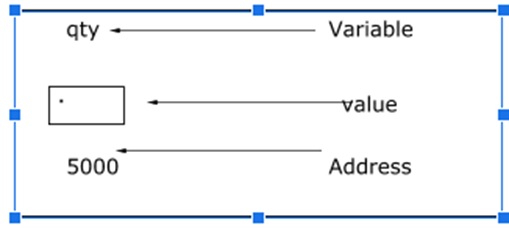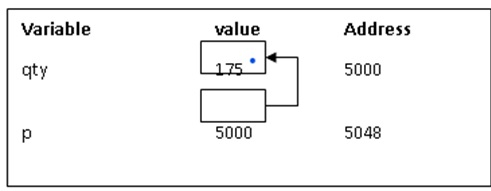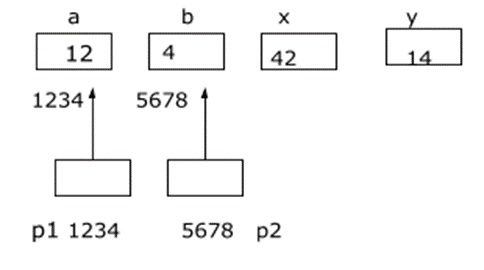# Explain Arithmetic operations using pointers in C language?

Pointer is a variable which stores the address of other variable.

## Pointer declaration, initialization and accessing

Consider the following statement −

int qty = 179;## Declaring a pointer

int *p;

‘p’ is a pointer variable that holds the address of another integer variable.

## Initialization of a pointer

Address operator (&) is used to initialize a pointer variable.

int qty = 175;
int *p;
p= &qty;## Arithmetic operations using pointers

Pointer variables can be used in expressions. For example, if pointer variables are properly declared and initialized then the following statements are valid.

a) *p1 + *p2
b) *p1- *p2
c) *p1 * *p2
d) *p1/ *p2 Note: There must be a blank space between / and *
otherwise it is treated as beginning of comment line
e ) p1 + 4
f) p2 - 2
g) p1 - p2 Note: returns the no. of elements in between p1 and p2 if
both of them point to same array
h) p1++
i) – – p2
j) sum + = *p2
j) p1 > p2
k) p1 = = p2
l) p1 ! = p2 Note: Comparisons can be used meaningfully in handling
arrays and strings

The following statements are invalid −

a) p1 + p2
b) p1 * p2
c) p1 / p2
d) p1 / 3

## Program

#include<stdio.h>
main (){
int a,b,x,y,z;
int *p1, *p2;
a =12;
b = 4;
p1= &a;
p2 = &b;
x = *p1 * * p2 – 6;
y= 4 - *p2 / *p1+10;
printf (“Address of a = %d”, p1);
printf (“Address of b = %d”, p2);
printf (“a= %d b =%d”, a,b);
printf (“x= %d y =%d”, x,y);
}

## Output

Address of a = 1234
x = 42 y= 14